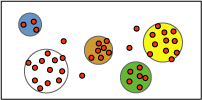# Microsoft Clustering Algorithm

The Microsoft Clustering algorithm is a segmentation algorithm provided by Microsoft SQL Server 2005 Analysis Services (SSAS). The algorithm uses iterative techniques to group cases in a dataset into clusters that contain similar characteristics. These groupings are useful for exploring data, identifying anomalies in the data, and creating predictions.

Clustering models identify relationships in a dataset that you might not logically derive through casual observation. For example, you can logically discern that people who commute to their jobs by bicycle do not typically live a long distance from where they work. The algorithm, however, can find other characteristics about bicycle commuters that are not as obvious. In the following diagram, cluster A represents data about people who tend to drive to work, while cluster B represents data about people who tend to ride bicycles to work.The clustering algorithm differs from other data mining algorithms, such as the Microsoft Decision Trees algorithm, in that you do not have to designate a predictable column to be able to build a clustering model. The clustering algorithm trains the model strictly from the relationships that exist in the data and from the clusters that the algorithm identifies.

## Example

Consider a group of people who share similar demographic information and who buy similar products from the Adventure Works company. This group of people represents a cluster of data. Several such clusters may exist in a database. By observing the columns that make up a cluster, you can more clearly see how records in a dataset are related to one another.

## How the Algorithm Works

The Microsoft Clustering algorithm first identifies relationships in a dataset and generates a series of clusters based on those relationships. A scatter plot is a useful way to visually represent how the algorithm groups data, as shown in the following diagram. The scatter plot represents all the cases in the dataset, and each case is a point on the graph. The clusters group points on the graph and illustrate the relationships that the algorithm identifies.After first defining the clusters, the algorithm calculates how well the clusters represent groupings of the points, and then tries to redefine the groupings to create clusters that better represent the data. The algorithm iterates through this process until it cannot improve the results more by redefining the clusters.

The Microsoft Clustering algorithm offers two methods for calculating how well points fit within the clusters: Expectation Maximization (EM) and K-Means. For EM clustering, the algorithm uses a probabilistic method to determine the probability that a data point exists in a cluster. For K-Means, the algorithm uses a distance measure to assign a data point to its closest cluster.

Columns with a usage that is set to predict only are not used to build clusters. Their distributions in the clusters are calculated after the clusters are built.

For a more detained explanation about how the Microsoft Clustering algorithm works, see Scaling EM (Expectation Maximization) Clustering to Large Databases.

## Using the Algorithm

A clustering model must contain a key column and input columns. You can also define input columns as being predictable.

The algorithm supports specific input column content types, predictable column content types, and modeling flags, which are listed in the following table.

 Input column content types Continuous, Cyclical, Discrete, Discretized, Key, Table, and Ordered Predictable column content types Continuous, Cyclical, Discrete, Discretized, Table, and Ordered Modeling flags MODEL_EXISTENCE_ONLY and NOT NULL

All Microsoft algorithms support a common set of functions. However, the Microsoft Clustering algorithm supports additional functions, listed in the following table.

For a list of the functions that are common to all Microsoft algorithms, see Data Mining Algorithms. For more information about how to use these functions, see Data Mining Extensions (DMX) Function Reference.

The Microsoft Clustering algorithm supports using the Predictive Model Markup Language (PMML) to create mining models.

The Microsoft Clustering algorithm supports several parameters that affect the performance and accuracy of the resulting mining model. The following table describes each parameter.

Parameter Description

CLUSTERING_METHOD

Specifies the clustering method for the algorithm to use. The following clustering methods are available: scalable EM (1), non-scalable EM (2), scalable K-Means (3), and non-scalable K-Means (4).

The default is 1.

CLUSTER_COUNT

Specifies the approximate number of clusters to be built by the algorithm. If the approximate number of clusters cannot be built from the data, the algorithm builds as many clusters as possible. Setting the CLUSTER_COUNT to 0 causes the algorithm to use heuristics to best determine the number of clusters to build.

The default is 10.

CLUSTER_SEED

Specifies the seed number that is used to randomly generate clusters for the initial stage of model building.

The default is 0.

MINIMUM_SUPPORT

Specifies the minimum number of cases in each cluster.

The default is 1.

MODELLING_CARDINALITY

Specifies the number of sample models that are constructed during the clustering process.

The default is 10.

STOPPING_TOLERANCE

Specifies the value that is used to determine when convergence is reached and the algorithm is finished building the model. Convergence is reached when the overall change in cluster probabilities is less than the ratio of the STOPPING_TOLERANCE parameter divided by the size of the model.

The default is 10.

SAMPLE_SIZE

Specifies the number of cases that the algorithm uses on each pass if the CLUSTERING_METHOD parameter is set to one of the scalable clustering methods. Setting the SAMPLE_SIZE parameter to 0 will cause the whole dataset to be clustered in a single pass. This can cause memory and performance issues.

The default is 50000.

MAXIMUM_INPUT_ATTRIBUTES

Specifies the maximum number of input attributes that the algorithm can handle before it invokes feature selection. Setting this value to 0 specifies that there is no maximum number of attributes.

The default is 255.

MAXIMUM_STATES

Specifies the maximum number of attribute states that the algorithm supports. If the number of states that an attribute has is larger than the maximum number of states, the algorithm uses the attribute’s most popular states and ignores the remaining states.

The default is 100.